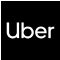New update is available. Click here to update.
Topics

# Search In A 2D Matrix

Easy0/40
Average time to solve is 10m+8 more companies

## Problem statement

You have been given a 2-D array 'mat' of size 'M x N' where 'M' and 'N' denote the number of rows and columns, respectively. The elements of each row are sorted in non-decreasing order.

Moreover, the first element of a row is greater than the last element of the previous row (if it exists).

You are given an integer ‘target’, and your task is to find if it exists in the given 'mat' or not.

Example:
``````Input: ‘M’ = 3, 'N' = 4, ‘mat’ = [[1, 2, 3, 4], [5, 6, 7, 8], [9, 10, 11, 12]], ‘target’ = 8

Output: true

Explanation: The output should be true as '8' exists in the matrix.
``````
Detailed explanation ( Input/output format, Notes, Images )
Sample Input 1 :
``````3 4 8
1 2 3 4
5 6 7 8
9 10 11 12
``````
Sample Output 1 :
``````true
``````
Explanation For Sample Input 1 :
``````The ‘target’  = 8 exists in the 'mat' at index (1, 3).
``````
Sample Input 2 :
``````3 3 78
1 2 4
6 7 8
9 10 34
``````
Sample Output 2 :
``````false
``````
Explanation For Sample Input 2 :
``````The ‘target' = 78 does not exist in the 'mat'. Therefore in the output, we see 'false'.
``````
Constraints :
``````1 <= N <= 50
1 <= M <= 50
-10^5 <= mat[i], target <= 10^5

Time Limit: 1 sec
``````Console